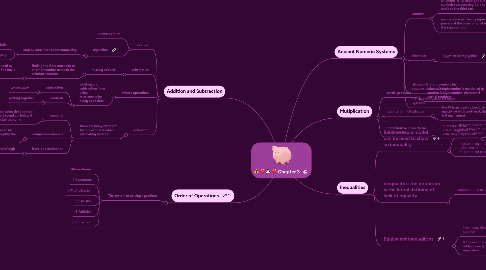# Chapter 3

Get Started. It's FreeChapter 3## 1. Inequalities

### 1.1. Balance scale model can be used to show an inequality

1.1.1. after the scale is "balanced" you can easily look at the picture of the items left over to find your answer!

1.1.2. to solve an equation is to find all replacements for the variable that makes the statement true.

### 1.2. inequalites: the definition is the literal defining of lack of equality.

1.2.1. statement that uses

1.2.1.1. less than (<)

1.2.1.2. is less than or equal to (< with a _ under it)

1.2.1.3. is greater than (>)

1.2.1.4. is greater than or equal to(> with a _under it)

1.2.1.5. or is not equal to (= with a slash through it)

### 1.3. Equivalent inequalities

1.3.1. two inequalities with the same solution

1.3.2. If the various problems add/subtract/multiply/or divide then it is equivalent

## 2. Ancient Numeric Systems

2.1.1. a number "b" is selected for a base and symbols representing 1,b,b to the second, and b to the third etc.

2.1.2. numbers are written by repeating the powers of the base for what ever number it is representing.

### 2.2. Example:

2.2.1. Egyptian hieroglyphics

2.2.1.1. can be written in any order

2.2.1.2. base ten system :b=10

2.2.1.4. ascending powers written from left to right

### 2.4. "0" was rarely used for most systems

3.1.1. known as "sum

3.1.2. algorithm

3.1.2.1. step by step method for computing

3.1.2.1.2. second step: regrouping or carrying

### 3.2. subtraction

3.2.1.1. finding the # we must add to another number to make the solution accurate

3.2.1.1.1. ex: 7-3 can be determined by finding what adds to 3 to make 7

### 3.3. inverse operations

3.3.1. addition and subtraction have a dual relationship by being opposites

3.3.1.1. subtraction

3.3.1.1.1. taking away

3.3.1.2.1. putting together

### 3.4. estimation

3.4.1. there are many different tecniques to use when estimating such as:

3.4.1.1. rounding

3.4.1.1.1. round up or down using the 5 system rule: if its above 5 round up, below 5 round down or stay the same

3.4.1.2. compatible numbers

3.4.1.2.1. when a number is relaced by another number to simplify the equation

3.4.1.3. front-end estimation

3.4.1.3.1. use only front end of digit

## 4. Mutiplication

### 4.1. partial products

4.1.1. when a 2 digit number is multiplied by another 2 digit number, there are 4 partial products.

### 4.2. identity for multiplication

4.2.1. the #1 is an identity because when multiplied with another #, the other # is left unchanged.

### 4.3. commutative property for multiplication

4.3.1. numbers can be interchanged without affecting the product

4.3.1.1. ex: a+b=b+a

## 5. Order of Operations

### 5.1. The system of solving a problem

5.1.1. 1)Parentheses

5.1.2. 2)Exponents

5.1.3. 3)Multiplication

5.1.4. 3)Division